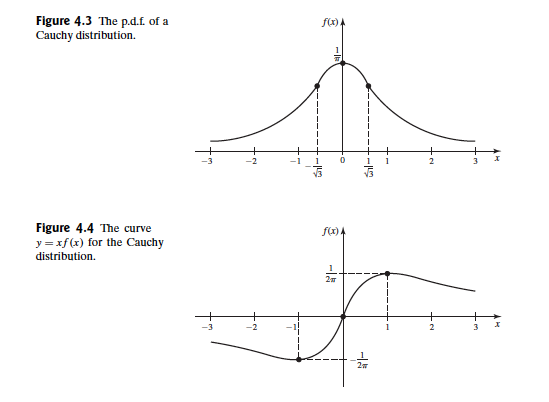Abstract: 本文主要介绍期望的基础之知识，第一部分介绍连续和离散随机变量的期望。
Keywords: Expectation

# 随机变量的期望

## 离散分布的期望 Expectation for a Discrete Distribution

Definition Mean of Bounded Discrete Random Variable. Let $X$ be a bounded discrete random variable whose p.f. is $f$ .the expectation of $X$ denoted by $E(X)$ ,is a number define as follow:
$$E(X)=\sum_{\text{All }x}xf(x)$$
The expectation of $X$ is also referred to as the mean of $X$ or the expected value of $X$

$$E(X)=p\times 1+(1-p)\times 0=p$$

Definition Mean of General Discrete Random Variable. Let X be a discrete random variable whose p.f. is f.Suppose that at least one of the following sums is finite:
$$\sum_{\text{Positive }x}xf(x) , \sum_{\text{Negative }x}xf(x)$$
Then the mean,expectation,or expected value of $X$ is said to exist and is defined to be
$$E(x)=\sum_{\text{All } x}xf(x)$$

$$f(x)= \begin{cases} \frac{1}{2|x|(|x|+1)}&\text{ if }x=\pm 1,\pm 2,\pm 3,\dots\\ 0& \text{ otherwise } \end{cases}$$

$$\sum^{-\infty}_{x=-1} x\frac{1}{2|x|(|x|+1)}=-\infty \\ \sum^{\infty}_{x=1} x\frac{1}{2|x|(|x|+1)}=\infty$$

## 连续分布的期望 Expectation for a Continuous Distribution

Definition Mean of Bounded Continuous Random Variable. Let X be a bounded continuous random variable whose p.d.f. is f.The expectation of X ,denote E(X),is defined as follows:
$$E(X)=\int^{\infty}_{-\infty}xf(x)dx$$

Definition Mean of General Continuous Random Variable.Let X be a continuous random variable whose p.d.f. is f.Suppose that at least one of the following integrals is finite:
$$\int^{\infty}_{0}xf(x)dx,\int^{0}_{-\infty}xf(x)dx$$
Then the mean,expectation,or expected value of X is said to exist and is defined to be
$$E(X)=\int^{\infty}_{-\infty}xf(x)dx$$

$$f(x)=\frac{1}{\pi(1+x^2)} \text{ for } -\infty < x < \infty$$

$$\frac{d}{dx}tan^{-1}(x)=\frac{1}{1+x^2}$$

$$\int^{\infty}_{0}\frac{x}{\pi(1+x^2)}dx=\infty$$

$$\int^{0}_{-\infty}\frac{x}{\pi(1+x^2)}dx=-\infty$$0%